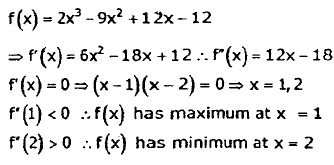Courses

# Engineering Mathematics And General Aptitude - 1

## 15 Questions MCQ Test Mock Test Series for Civil Engineering (CE) GATE 2020 | Engineering Mathematics And General Aptitude - 1

Description
This mock test of Engineering Mathematics And General Aptitude - 1 for GATE helps you for every GATE entrance exam. This contains 15 Multiple Choice Questions for GATE Engineering Mathematics And General Aptitude - 1 (mcq) to study with solutions a complete question bank. The solved questions answers in this Engineering Mathematics And General Aptitude - 1 quiz give you a good mix of easy questions and tough questions. GATE students definitely take this Engineering Mathematics And General Aptitude - 1 exercise for a better result in the exam. You can find other Engineering Mathematics And General Aptitude - 1 extra questions, long questions & short questions for GATE on EduRev as well by searching above.
QUESTION: 1

### The dimension of a pressure gradient in a fluid flow are :

Solution:

Pressure gradient= Pressure per unit length
Pressure= Force/ Area= (Mass x Acceleration)/Area =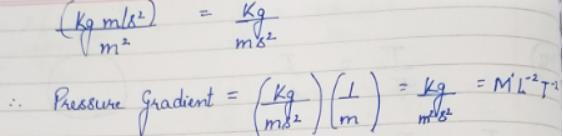QUESTION: 2

### The following Newton-Raphson iteration formula finds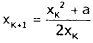Solution: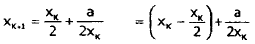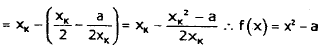QUESTION: 3

### Find the 2nd approximation to the root of the equation t4 -4t-9 = 0 between 2 and 2.5 using Bisection method.

Solution: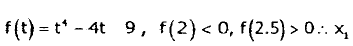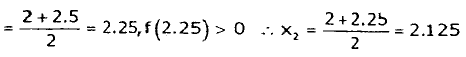QUESTION: 4

Consider two sets of vectors P and Q as

P = {(2,-1,3,2), (1,3,4,2), (3,-5,2,2)}
Q = {(.1,2,-1,0), (1,3,1,2), (4,2,1,0), (6,1,0,1)}

Which of the following is true?

Solution: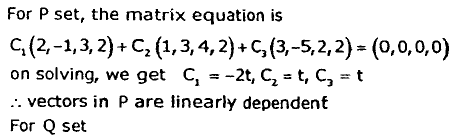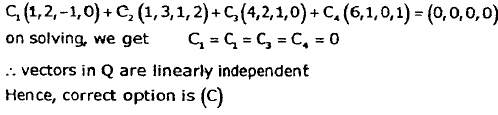QUESTION: 5

Let G1 and G2 be two edge disjoint and vertex disjoint graphs. G1 has 4 vertices and 6 edges, G2 has 6 vertices and 10 edges. Then number of edges in (G1 intersection G2) and (G1 union G2) are respectively

Solution:

Edge disjoint means no edge is common and vertex disjoint means no vertex is common.
As there is no edge is common between G1 and G2, hence number of edges in (G1 intersection G2) = 0 and number of edges in (G1 union G2) = number of edges in G1 + number of edges in G2 = 6+10 = 16

QUESTION: 6

Which of the following propositions is a tautology?

Solution:
*Answer can only contain numeric values
QUESTION: 7

The minimum number of connected components of a simple graph with 20 vertices and 12 edges is

Solution:

e: number of edges = 12, n: number of vertices = 20
k: number of connected components, e > n - k , Or, 12 > 20-K ⇒ K = 8

QUESTION: 8

Which of the following graphs has a Hamiltonian circuit?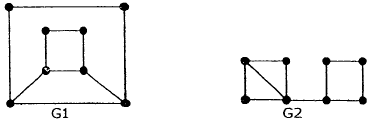Solution: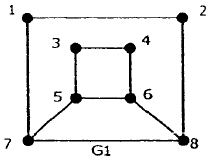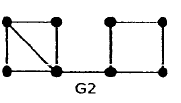G1 has a Hamiltonian circuit: 1-2-8-6-4-3-5-7-1
G2 has a bridge (an edge which, if removed, disconnects the graph), namely the middle edge of the thcee horizontal edges at the bottom of the given .drawing of G2. Whichever side of the bridge you start, you cannot, having crossed the bridge, re-cross it without visiting vertices twice. Hence you cannot complete a Hamiltonian circuit.

QUESTION: 9

The proposition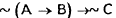logically equivalent to

Solution: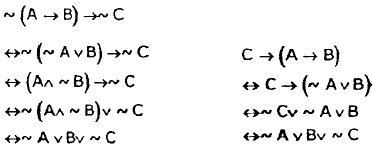QUESTION: 10

Investigate the values of λ and μ so that the equations

2x+3y+5z = 9, 7x+3y-2z = 8, 2x+3y+λ z = μ

have an infinite number of solutions.

Solution: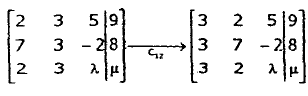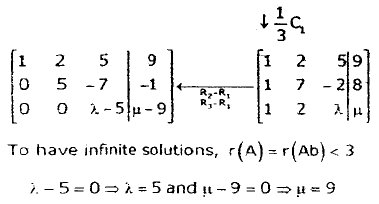QUESTION: 11

A complex (P+ iQ, P,Q : real numbers) n x n matrix A is said to be unitary if AA° = In, where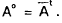If A be a unitary matrix, then which of the following is true?

Solution: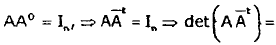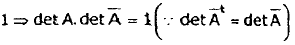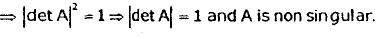*Answer can only contain numeric values
QUESTION: 12

Consider the function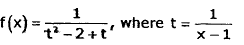At how many points, f(x) Is discontinuous?

Solution: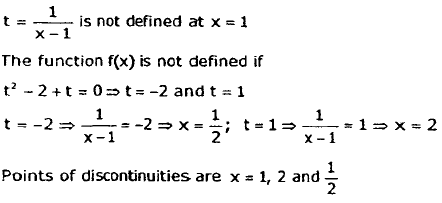QUESTION: 13

Evaluate the integral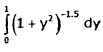Solution: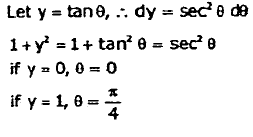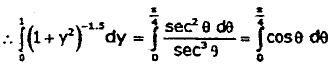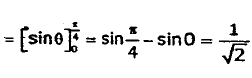QUESTION: 14

Consider the following matrix: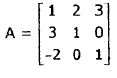Determine the eigen values of the given matrix.

Solution: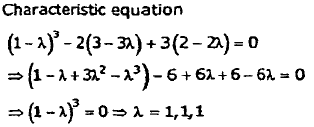QUESTION: 15

Consider the function f (x) = 2x3 - 9x2 + 12x -12

The function attains minima at x =

Solution: